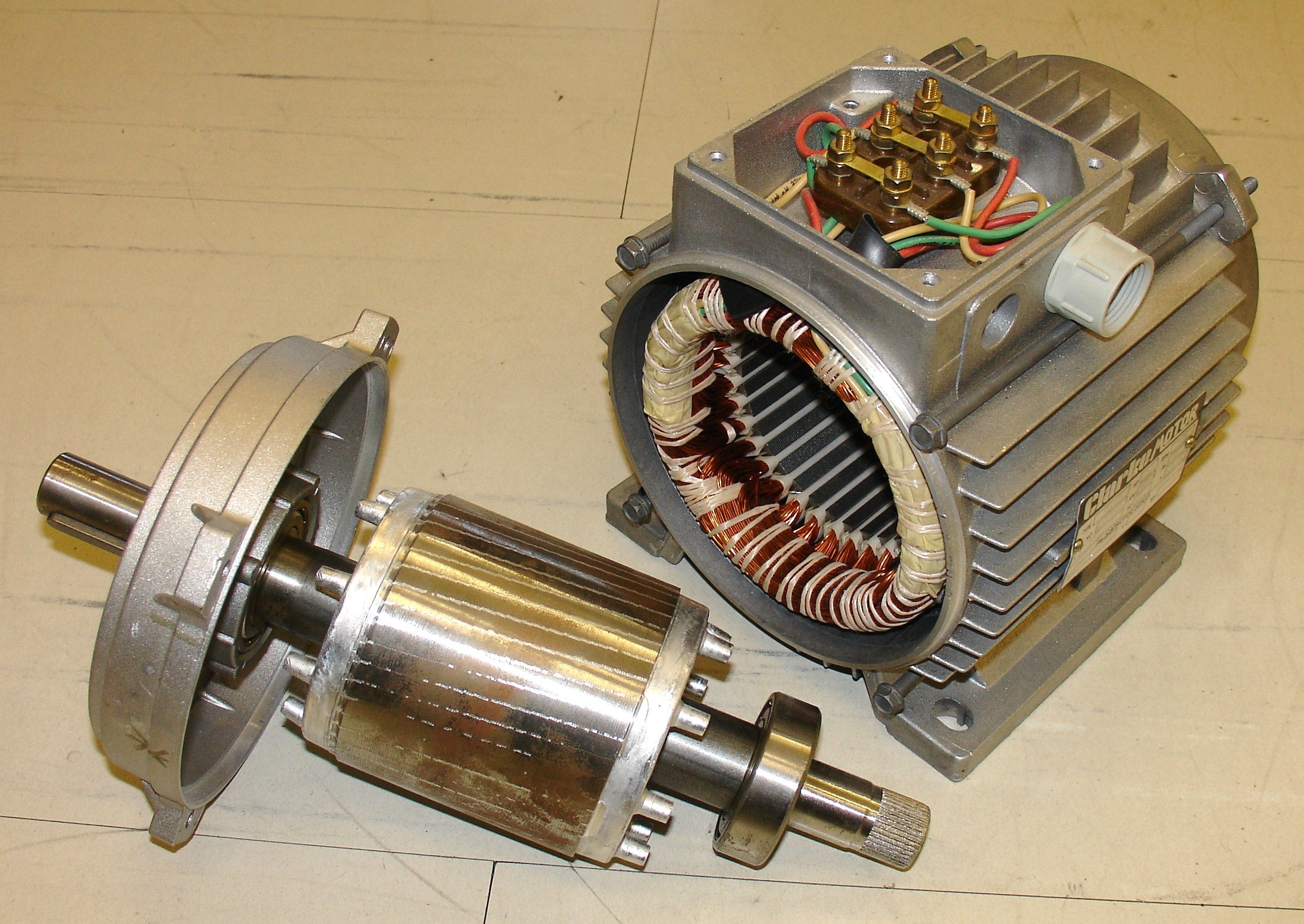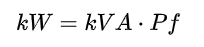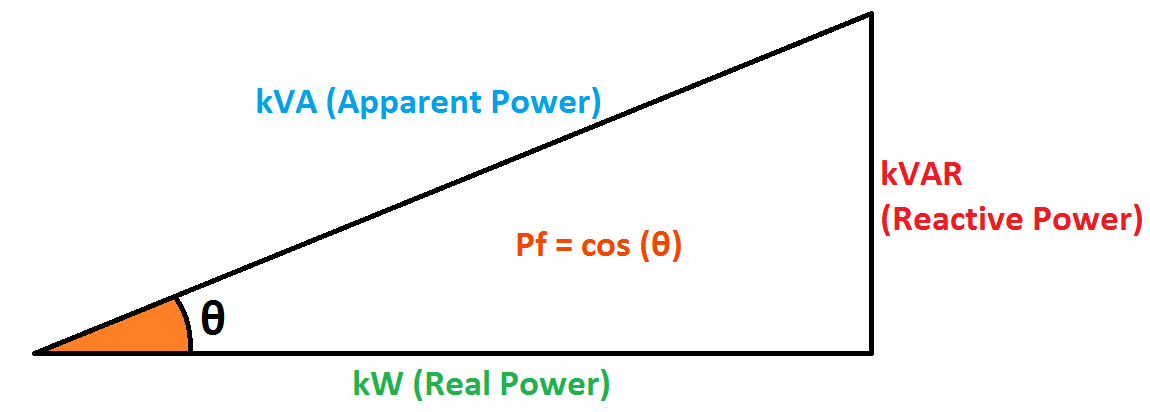Following the successful completion of this module, you will be able to:

• Understand how a motor works on a basic level.
• Identify the main components of a motor.
• Understand how power is used in a motor.
• Understand the differences between induction motors, synchronous motors, and DC motors.
• Calculate the current operating efficiency of a motor.
• Understand where the power losses occur in a motor.
• Understand the types of loads that appear in an industrial setting.
• Recognize the various types of mechanical motor speed control technologies.
• Recognize the main types of electronic motor speed control technologies.

### Fundamentals

#### Electromechanical Energy Conversion

"The twisting force (torque) applied to the shaft is produced by the interaction of two magnetic fields, one produced by the fixed part of the motor (stator) and the other produced by the rotating component of the motor (rotor). The forces developed in a motor resemble the force between two magnets held close together: similar poles repel each other, dissimilar poles attract. If one of the magnets is mounted on a shaft, the attracting and repelling forces create torque." (Energy-Efficient Motor Systems, 2002).

#### Motor Main Components

The Stator is attached to the frame of the motor and generates a magnetic field from its windings energized by the supplied electricity.

The Rotor is attached to the output shaft and generates a magnetic field from its windings that interacts with the stator's magnetic field, producing torque.

The Common Industrial Motor Types module explains how a magnetic field can be created from the stator and rotor.Construction of an Induction Motor (Wikipedia)  Rotor on left side, Stator on right side

#### Power

Motors convert electric power into mechanical power, but not all the power received by the motor is converted to mechanical energy. A fraction of all the energy "apparently" consumed by the motor is used to generate the magnetic fields that put the motor into motion. This is called Reactive Power, which is measured in kVAR (Kilovolt-Amperes Reactive). This power is not converted to mechanical energy. If the Total Power (Kilovolt-Amperes) and Power Factor of a motor is known, the actual power being converted to mechanical power can be calculated:Total Power consists of Real Power (kW) and Reactive Power (kVA). The Power Triangle describes the relationship between the powers and its effect on Power Factor (Pf):The real power being consumed will also include any motor energy losses that will be explained in the Efficiency module.

Reactive Power is undesirable because the motor is essentially absorbing power from the grid and delivering it back. This causes extra load on the utility provider, and many companies charge fees for low power factor and/or excessive reactive power.

Capacitor banks can be installed at industrial facilities to reduce the amount of reactive power consumed and is a potential energy savings recommendation.